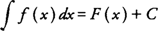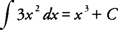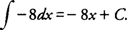## Antiderivatives/Indefinite Integrals

A function F( x) is called an antiderivative of a function of f( x) if F′( x) = f( x) for all x in the domain of f. Note that the function F is not unique and that an infinite number of antiderivatives could exist for a given function. For example, F( x) = x 3, G( x) = x 3 + 5, and H( x) = x 3 − 2 are all antiderivatives of f( x) = 3 x 2 because F′( x) = G′( x) = H′( x) = f( x) for all x in the domain of f. It is clear that these functions F, G, and H differ only by some constant value and that the derivative of that constant value is always zero. In other words, if F( x) and G( x) are antiderivatives of f( x) on some interval, then F′( x) = G′( x) and F( x) = G( x) + C for some constant C in the interval. Geometrically, this means that the graphs of F( x) and G( x) are identical except for their vertical position.

The notation used to represent all antiderivatives of a function f( x) is the indefinite integral symbol written, where. The function of f( x) is called the integrand, and C is reffered to as the constant of integration. The expression F( x) + C is called the indefinite integral of F with respect to the independent variable x. Using the previous example of F( x) = x 3 and f( x) = 3 x 2, you find that.

The indefinite integral of a function is sometimes called the general antiderivative of the function as well.

Example 1: Find the indefinite integral of f( x) = cos xExample 2: Find the general antiderivative of f( x) = –8.

• Because the derivative of F( x) = −8 x is F′( x) = −8, write